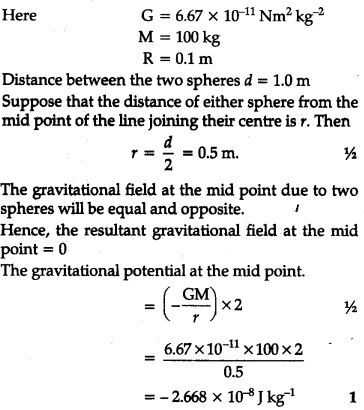# Two heavy spheres each of mass 100 kg and radius 0.10 m are placed 1.0 m apart on a horizontal table

Two heavy spheres each of mass 100 kg and radius 0.10 m are placed 1.0 m apart on a horizontal table. What is the gravitational force and potential at the mid point of the line joining the centres of the spheres. Is an object placed at the point is in equilibrium ? If so, is the equilibrium stable or unstable ?